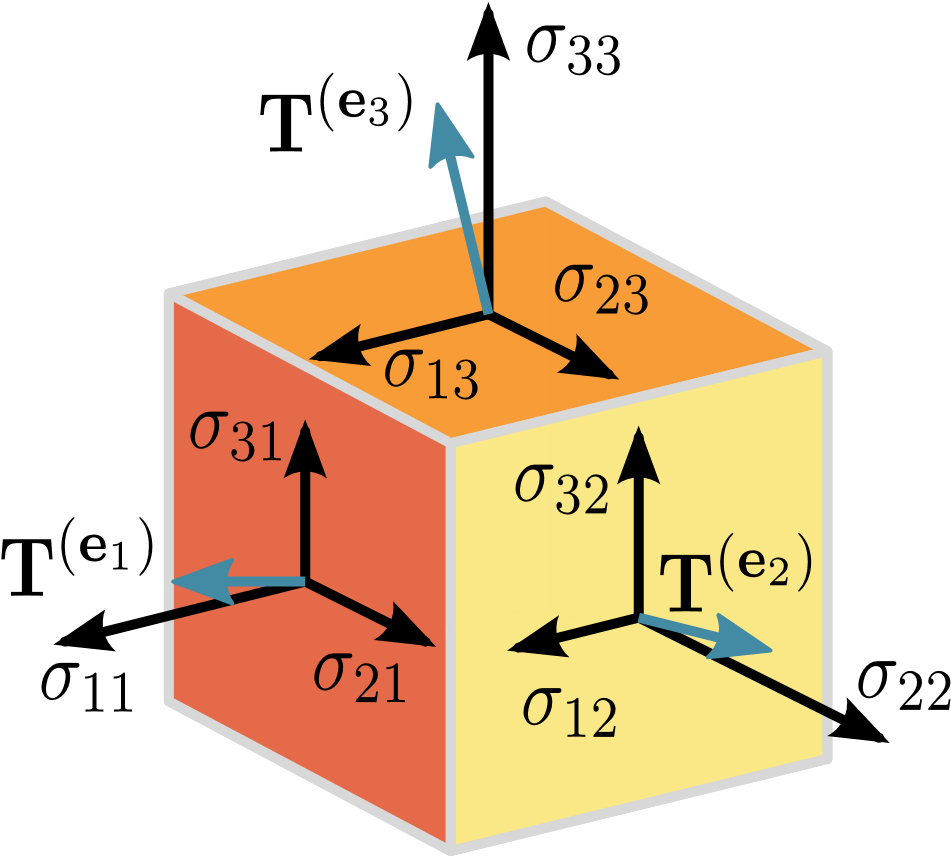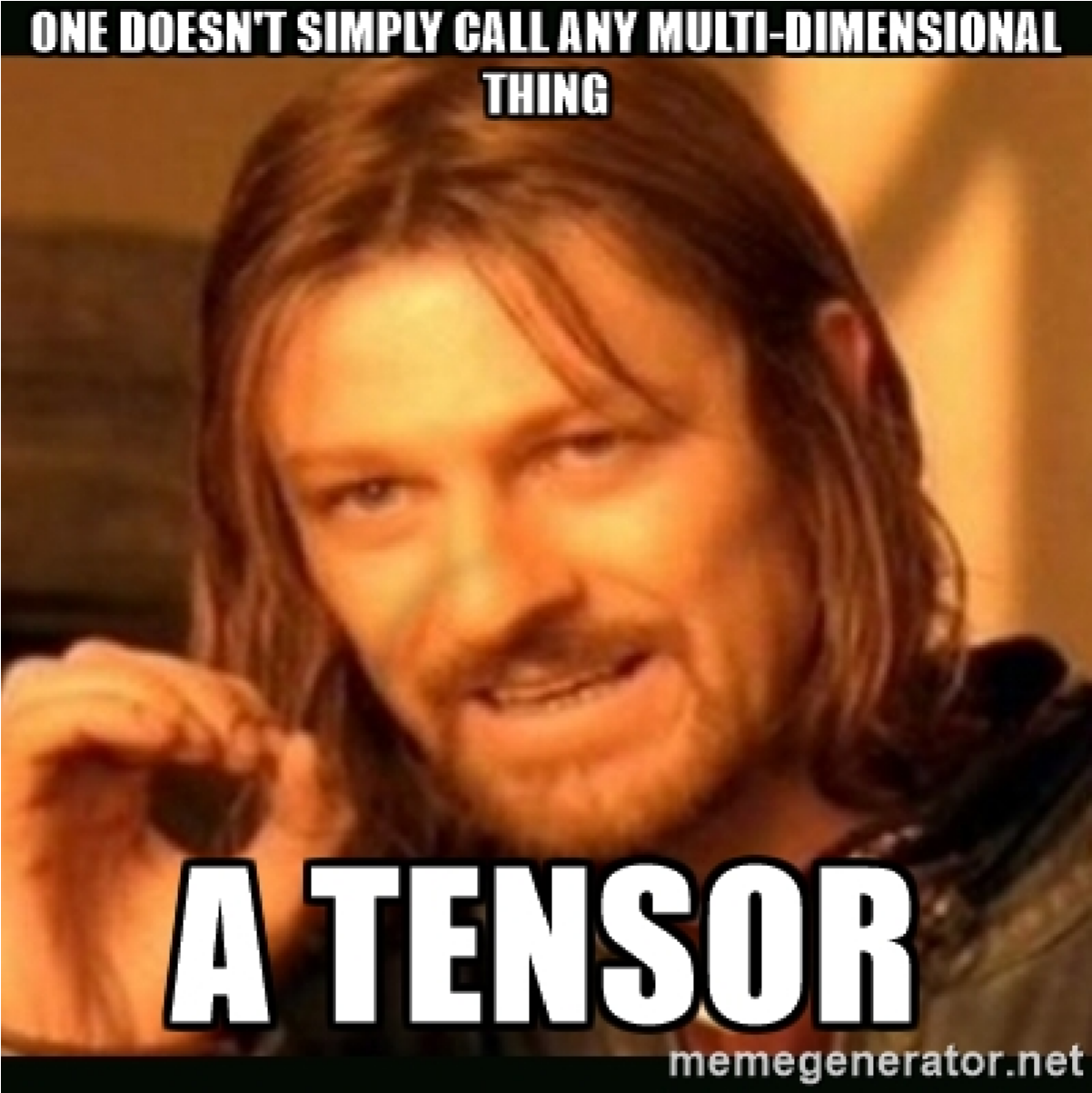Home » TensorFlow 101: Understanding Tensors and Graphs to get you started in Deep Learning

# TensorFlow 101: Understanding Tensors and Graphs to get you started in Deep Learning

## Introduction

TensorFlow is one of the most popular libraries in Deep Learning. When I started with TensorFlow it felt like an alien language. But after attending couple of sessions in TensorFlow, I got the hang of it. I found the topic so interesting that I delved further into it.

While reading about TensorFlow, I understood one thing. In order to understand TensorFlow one needs to understand Tensors and Graphs. These are two basic things Google tried to incorporate in it’s Deep Learning framework.

## What are Tensors?As per the wiki definition of Tensors:

Tensors are geometric objects that describe linear relations between geometric vectors, scalars, and other tensors. Elementary examples of such relations include the dot product, the cross product, and linear maps. Geometric vectors, often used in physics and engineering applications, and scalars themselves are also tensors.

As the definition goes, Deep Learning wants us to think that Tensors as Multidimensional Arrays.

In a recent talk by one of my colleagues, he was required to show the difference between a Neural Network made in NumPy and Tensors. While creating the material for the talk, he observed that NumPy and Tensors take almost the same time to run (with different optimizers).

We both banged our headache over it in order to prove TensorFlow is better but we couldn’t. This kept disturbing me and I decided to delve further into it.

Now, we need to understand Tensors and NumPy first.

As per the NumPy official website, it says:

NumPy can also be used as an efficient multi­dimensional container of generic data. Arbitrary data­types can be defined. This allows NumPy to seamlessly and speedily integrate with a wide variety of databases.

After reading this I’m sure the same question must have popped in your head as in mine. What’s the difference between Tensors and N­Dimensional Arrays?As per Stackexchange,  Tensor : Multidimensional array :: Linear transformation : Matrix.

The above expression means tensors and multidimensional arrays are different types of object. The first is a type of function, the second is a data structure suitable for representing a tensor in a coordinate system.

Mathematically, tensors are defined as a multilinear function. A multi-linear function consists of various vector variables. A tensor field is a tensor ­valued function. For a rigorous mathematical explanation you can read here.

Which means tensors are functions or containers which we need to define. The actual calculation happens when there’s data fed.  What we see as NumPy arrays (1D, 2D, …, ND) can be considered as generic tensors.

I hope now you would have some understanding of what are Tensors.

## Why we need Tensors in TensorFlow?

Now, the big questions is why we need to deal with Tensors in Tensorflow. The big revelation is what NumPy lacks is creating Tensors. We can convert tensors to NumPy and vice­versa. That is possible since the constructs are defined definitely as arrays/matrices.

I could get a few answers reading and searching for Tensors and NumPy arrays. For more reading, there’s no better resources than the official documentations.

## What are Graphs?

Theano’s meta­-programming structure seems to be an inspiration for Google to create Tensorflow, but folks at Google took it to a next level.

According to the official Tensorflow blog on Getting Started.

A computational graph is a series of TensorFlow operations arranged into a graph of nodes.

```import tensorflow as tf
# If we consider a simple multiplication a = 2 b = 3 mul = a*b
print ("The multiplication produces:::", mul)
The multiplication produces::: 6
# But consider a tensorflow program to replicate above at = tf.constant(3) bt = tf.constant(4)
mult = tf.mul(at, bt)
print ("The multiplication produces:::", mult)
The multiplication produces::: Tensor("Mul:0", shape=(), dtype=int32)```

Each node takes zero or more tensors as inputs and produces a tensor as an output. One type of node is a constant. Like all TensorFlow constants, it takes no inputs, and it outputs a value it stores internally.

I think the above statement holds true as we have seen that constructing a computational graph to multiply two values is rather a straight forward task. But we need the value at the end. We have defined the two constants, at and bt, along with their values. What if we don’t define the values?

Let’s check:

```at = tf.constant() bt
= tf.constant()
mult = tf.mul(at, bt)
print ("The multiplication produces:::", mult)
‐‐‐‐‐‐‐‐‐‐‐‐‐‐‐‐‐‐‐‐‐‐‐‐‐‐‐‐‐‐‐‐‐‐‐‐‐‐‐‐‐‐‐‐‐
‐‐‐‐‐‐‐‐‐‐‐‐‐‐‐‐‐‐‐‐‐‐‐‐‐‐‐‐‐‐
TypeError Traceback (most recent call last)
<ipython‐input‐3‐3d0aff390325> in <module>()
‐‐‐‐> 1 at = tf.constant()
2 bt = tf.constant()
3
4 mult = tf.mul(at, bt)
5```
`TypeError: constant() missing 1 required positional argument: 'value'`

I guess the constant needs a value. Next step would be to find out why we didn’t get any output. It seems that to evaluate the graph that we made, it needs to be run in a session.

To understand this complexity, we need to understand what our computational graph has:

• Tensors: at, bt
• Operations: mult

To execute mult, the computational graph needs a session where the tensors and operations would be evaluated. Let’s now evaluate our graph in a session.

`sess = tf.Session()`
```# Executing the session
print ("The actual multiplication result:::", sess.run(mult))
The actual multiplication result::: 12```

The above graph would print the same value since we are using constants. There are 2 more ways we could send values to the graph ­- Variables and Placeholders.

### Variables

When you train a model, you use variables to hold and update parameters. Variables are in memory buffers containing tensors. They must be explicitly initialized and can be saved to disk during and after training. You can later restore saved values to exercise or analyze the model.

Variable initializers must be run explicitly before other ops in your model can run. The easiest way to do that is to add an op that runs all the variable initializers, and run that op before using the model.

We can initialize variables from another variables too.  Constants can’t be updated, that’s a shame everywhere. Need to check whether dynamically variables can be created.

We can conclude that placeholders is a way to define variables without actually defining the values to be passed to it when we create a computational graph.

tf.placeholder() is the norm, used by all the Tensorflow folks writing code daily.

For a more in depth reading: I/O for Tensorflow.

We would check out Variables and Placeholders below.

```# Variable

var1 = tf.Variable(2, name="var1") var2
= tf.Variable(3, name="var2")
mulv = tf.mul(var1, var2)
print (mulv)

Tensor("Mul_2:0", shape=(), dtype=int32)
with tf.Session() as sess:
sess.run(tf.global_variables_initializer()) # always need to initialize the variable
print ("The variable var1 is:::", sess.run(var1))
print ("The variable var2 is:::", sess.run(var2))
print ("The computational result is:::", sess.run(mulv))```
```The variable var1 is::: 2
The variable var2 is::: 3
The computational result is::: 6```

```# Placeholder

pl = tf.placeholder(tf.float32, name="p")
pi = tf.constant(3.) c = tf.add(pl, pi)
print (c)

with tf.Session() as sess:
sess.run(tf.global_variables_initializer()) # always need to initialize the var iables
writer = tf.train.SummaryWriter("output", sess.graph)
#print("The placeholder value passed:::", sess.run(pl, {pl:3}))
print("The calculation result is:::", sess.run(c, {pl:3}))
writer.close()
WARNING:tensorflow:From <ipython‐input‐15‐4c5578691c20>:3 in <module>.: SummaryWriter.__init__ (from tensorflow.python.training.summary_io) is deprecated and will be
removed after 2016‐11‐30.
Instructions for updating:
Please switch to tf.summary.FileWriter. The interface and behavior is the same; thi s is just a rename.
The calculation result is::: 6.0```

### End Notes

In this article, we observed the  basics of Tensors and what do these do in a computational graph. The actual objective for creating this is to make Tensors flow through the graph. We write the tensors and through sessions we make them flow.

I hope you enjoyed reading this article.If you have any questions or doubts feel free to post them below.

### References

1. Tensorflow ­ Getting Started
2. CS224d
3. MetaFlow Blog
4. Theano vs Tensorflow
5. Machine Learning with TensorflowPrathamesh Sarang works as a Data Scientist at Lemoxo Technologies. Data Engineering is his latest love, turned towards the *nix faction recently. Strong advocate of “Markdown for everyone

By Analytics Vidhya Team: This article was contributed by Pratham Sarang who is the third rank holder of  Blogathon 3.

### Learn, compete, hack and get hired!

You can also read this article on our Mobile APP•Sachin shanbhag says: